# NumPy: the absolute basics for beginners¶

Welcome to the absolute beginner’s guide to NumPy! If you have comments or suggestions, please don’t hesitate to reach out!

## Welcome to NumPy!¶

NumPy (Numerical Python) is an open source Python library that’s used in almost every field of science and engineering. It’s the universal standard for working with numerical data in Python, and it’s at the core of the scientific Python and PyData ecosystems. NumPy users include everyone from beginning coders to experienced researchers doing state-of-the-art scientific and industrial research and development. The NumPy API is used extensively in Pandas, SciPy, Matplotlib, scikit-learn, scikit-image and most other data science and scientific Python packages.

The NumPy library contains multidimensional array and matrix data structures (you’ll find more information about this in later sections). It provides ndarray, a homogeneous n-dimensional array object, with methods to efficiently operate on it. NumPy can be used to perform a wide variety of mathematical operations on arrays. It adds powerful data structures to Python that guarantee efficient calculations with arrays and matrices and it supplies an enormous library of high-level mathematical functions that operate on these arrays and matrices.

## Installing NumPy¶

To install NumPy, we strongly recommend using a scientific Python distribution. If you’re looking for the full instructions for installing NumPy on your operating system, you can find all of the details here.

If you already have Python, you can install NumPy with:

```conda install numpy
```

or

```pip install numpy
```

If you don’t have Python yet, you might want to consider using Anaconda. It’s the easiest way to get started. The good thing about getting this distribution is the fact that you don’t need to worry too much about separately installing NumPy or any of the major packages that you’ll be using for your data analyses, like pandas, Scikit-Learn, etc.

You can find all of the installation details in the Installation section at SciPy.

## How to import NumPy¶

Any time you want to use a package or library in your code, you first need to make it accessible.

In order to start using NumPy and all of the functions available in NumPy, you’ll need to import it. This can be easily done with this import statement:

```import numpy as np
```

(We shorten `numpy` to `np` in order to save time and also to keep code standardized so that anyone working with your code can easily understand and run it.)

If you aren’t already comfortable with reading tutorials that contain a lot of code, you might not know how to interpret a code block that looks like this:

```>>> a = np.arange(6)
>>> a2 = a[np.newaxis, :]
>>> a2.shape
(1, 6)
```

If you aren’t familiar with this style, it’s very easy to understand. If you see `>>>`, you’re looking at input, or the code that you would enter. Everything that doesn’t have `>>>` in front of it is output, or the results of running your code. This is the style you see when you run `python` on the command line, but if you’re using IPython, you might see a different style.

## What’s the difference between a Python list and a NumPy array?¶

NumPy gives you an enormous range of fast and efficient ways of creating arrays and manipulating numerical data inside them. While a Python list can contain different data types within a single list, all of the elements in a NumPy array should be homogenous. The mathematical operations that are meant to be performed on arrays would be extremely inefficient if the arrays weren’t homogenous.

Why use NumPy?

NumPy arrays are faster and more compact than Python lists. An array consumes less memory and is convenient to use. NumPy uses much less memory to store data and it provides a mechanism of specifying the data types. This allows the code to be optimized even further.

## What is an array?¶

An array is a central data structure of the NumPy library. An array is a grid of values and it contains information about the raw data, how to locate an element, and how to interpret an element. It has a grid of elements that can be indexed in various ways. The elements are all of the same type, referred to as the array `dtype`.

An array can be indexed by a tuple of nonnegative integers, by booleans, by another array, or by integers. The `rank` of the array is the number of dimensions. The `shape` of the array is a tuple of integers giving the size of the array along each dimension.

One way we can initialize NumPy arrays is from Python lists, using nested lists for two- or higher-dimensional data.

For example:

```>>> a = np.array([1, 2, 3, 4, 5, 6])
```

or:

```>>> a = np.array([[1, 2, 3, 4], [5, 6, 7, 8], [9, 10, 11, 12]])
```

We can access the elements in the array using square brackets. When you’re accessing elements, remember that indexing in NumPy starts at 0. That means that if you want to access the first element in your array, you’ll be accessing element “0”.

```>>> print(a)
[1 2 3 4]
```

This section covers `1D array`, `2D array`, `ndarray`, `vector`, `matrix`

You might occasionally hear an array referred to as a “ndarray,” which is shorthand for “N-dimensional array.” An N-dimensional array is simply an array with any number of dimensions. You might also hear 1-D, or one-dimensional array, 2-D, or two-dimensional array, and so on. The NumPy `ndarray` class is used to represent both matrices and vectors. A vector is an array with a single dimension (there’s no difference between row and column vectors), while a matrix refers to an array with two dimensions. For 3-D or higher dimensional arrays, the term tensor is also commonly used.

What are the attributes of an array?

An array is usually a fixed-size container of items of the same type and size. The number of dimensions and items in an array is defined by its shape. The shape of an array is a tuple of non-negative integers that specify the sizes of each dimension.

In NumPy, dimensions are called axes. This means that if you have a 2D array that looks like this:

```[[0., 0., 0.],
[1., 1., 1.]]
```

Your array has 2 axes. The first axis has a length of 2 and the second axis has a length of 3.

Just like in other Python container objects, the contents of an array can be accessed and modified by indexing or slicing the array. Unlike the typical container objects, different arrays can share the same data, so changes made on one array might be visible in another.

Array attributes reflect information intrinsic to the array itself. If you need to get, or even set, properties of an array without creating a new array, you can often access an array through its attributes.

## How to create a basic array¶

This section covers `np.array()`, `np.zeros()`, `np.ones()`, `np.empty()`, `np.arange()`, `np.linspace()`, `dtype`

To create a NumPy array, you can use the function `np.array()`.

All you need to do to create a simple array is pass a list to it. If you choose to, you can also specify the type of data in your list. You can find more information about data types here.

```>>> import numpy as np
>>> a = np.array([1, 2, 3])
```

You can visualize your array this way:Be aware that these visualizations are meant to simplify ideas and give you a basic understanding of NumPy concepts and mechanics. Arrays and array operations are much more complicated than are captured here!

Besides creating an array from a sequence of elements, you can easily create an array filled with `0`’s:

```>>> np.zeros(2)
array([0., 0.])
```

Or an array filled with `1`’s:

```>>> np.ones(2)
array([1., 1.])
```

Or even an empty array! The function `empty` creates an array whose initial content is random and depends on the state of the memory. The reason to use `empty` over `zeros` (or something similar) is speed - just make sure to fill every element afterwards!

```>>> # Create an empty array with 2 elements
>>> np.empty(2)
array([ 3.14, 42.  ])  # may vary
```

You can create an array with a range of elements:

```>>> np.arange(4)
array([0, 1, 2, 3])
```

And even an array that contains a range of evenly spaced intervals. To do this, you will specify the first number, last number, and the step size.

```>>> np.arange(2, 9, 2)
array([2, 4, 6, 8])
```

You can also use `np.linspace()` to create an array with values that are spaced linearly in a specified interval:

```>>> np.linspace(0, 10, num=5)
array([ 0. ,  2.5,  5. ,  7.5, 10. ])
```

While the default data type is floating point (`np.float64`), you can explicitly specify which data type you want using the `dtype` keyword.

```>>> x = np.ones(2, dtype=np.int64)
>>> x
array([1, 1])
```

## Adding, removing, and sorting elements¶

This section covers `np.sort()`, `np.concatenate()`

Sorting an element is simple with `np.sort()`. You can specify the axis, kind, and order when you call the function.

```>>> arr = np.array([2, 1, 5, 3, 7, 4, 6, 8])
```

You can quickly sort the numbers in ascending order with:

```>>> np.sort(arr)
array([1, 2, 3, 4, 5, 6, 7, 8])
```

In addition to sort, which returns a sorted copy of an array, you can use:

To read more about sorting an array, see: `sort`.

```>>> a = np.array([1, 2, 3, 4])
>>> b = np.array([5, 6, 7, 8])
```

You can concatenate them with `np.concatenate()`.

```>>> np.concatenate((a, b))
array([1, 2, 3, 4, 5, 6, 7, 8])
```

```>>> x = np.array([[1, 2], [3, 4]])
>>> y = np.array([[5, 6]])
```

You can concatenate them with:

```>>> np.concatenate((x, y), axis=0)
array([[1, 2],
[3, 4],
[5, 6]])
```

In order to remove elements from an array, it’s simple to use indexing to select the elements that you want to keep.

To read more about concatenate, see: `concatenate`.

## How do you know the shape and size of an array?¶

This section covers `ndarray.ndim`, `ndarray.size`, `ndarray.shape`

`ndarray.ndim` will tell you the number of axes, or dimensions, of the array.

`ndarray.size` will tell you the total number of elements of the array. This is the product of the elements of the array’s shape.

`ndarray.shape` will display a tuple of integers that indicate the number of elements stored along each dimension of the array. If, for example, you have a 2-D array with 2 rows and 3 columns, the shape of your array is `(2, 3)`.

For example, if you create this array:

```>>> array_example = np.array([[[0, 1, 2, 3],
...                            [4, 5, 6, 7]],
...
...                           [[0, 1, 2, 3],
...                            [4, 5, 6, 7]],
...
...                           [[0 ,1 ,2, 3],
...                            [4, 5, 6, 7]]])
```

To find the number of dimensions of the array, run:

```>>> array_example.ndim
3
```

To find the total number of elements in the array, run:

```>>> array_example.size
24
```

And to find the shape of your array, run:

```>>> array_example.shape
(3, 2, 4)
```

## Can you reshape an array?¶

This section covers `arr.reshape()`

Yes!

Using `arr.reshape()` will give a new shape to an array without changing the data. Just remember that when you use the reshape method, the array you want to produce needs to have the same number of elements as the original array. If you start with an array with 12 elements, you’ll need to make sure that your new array also has a total of 12 elements.

```>>> a = np.arange(6)
>>> print(a)
[0 1 2 3 4 5]
```

You can use `reshape()` to reshape your array. For example, you can reshape this array to an array with three rows and two columns:

```>>> b = a.reshape(3, 2)
>>> print(b)
[[0 1]
[2 3]
[4 5]]
```

With `np.reshape`, you can specify a few optional parameters:

```>>> numpy.reshape(a, newshape=(1, 6), order='C')
array([[0, 1, 2, 3, 4, 5]])
```

`a` is the array to be reshaped.

`newshape` is the new shape you want. You can specify an integer or a tuple of integers. If you specify an integer, the result will be an array of that length. The shape should be compatible with the original shape.

`order:` `C` means to read/write the elements using C-like index order, `F` means to read/write the elements using Fortran-like index order, `A` means to read/write the elements in Fortran-like index order if a is Fortran contiguous in memory, C-like order otherwise. (This is an optional parameter and doesn’t need to be specified.)

If you want to learn more about C and Fortran order, you can read more about the internal organization of NumPy arrays here. Essentially, C and Fortran orders have to do with how indices correspond to the order the array is stored in memory. In Fortran, when moving through the elements of a two-dimensional array as it is stored in memory, the first index is the most rapidly varying index. As the first index moves to the next row as it changes, the matrix is stored one column at a time. This is why Fortran is thought of as a Column-major language. In C on the other hand, the last index changes the most rapidly. The matrix is stored by rows, making it a Row-major language. What you do for C or Fortran depends on whether it’s more important to preserve the indexing convention or not reorder the data.

## How to convert a 1D array into a 2D array (how to add a new axis to an array)¶

This section covers `np.newaxis`, `np.expand_dims`

You can use `np.newaxis` and `np.expand_dims` to increase the dimensions of your existing array.

Using `np.newaxis` will increase the dimensions of your array by one dimension when used once. This means that a 1D array will become a 2D array, a 2D array will become a 3D array, and so on.

```>>> a = np.array([1, 2, 3, 4, 5, 6])
>>> a.shape
(6,)
```

You can use `np.newaxis` to add a new axis:

```>>> a2 = a[np.newaxis, :]
>>> a2.shape
(1, 6)
```

You can explicitly convert a 1D array with either a row vector or a column vector using `np.newaxis`. For example, you can convert a 1D array to a row vector by inserting an axis along the first dimension:

```>>> row_vector = a[np.newaxis, :]
>>> row_vector.shape
(1, 6)
```

Or, for a column vector, you can insert an axis along the second dimension:

```>>> col_vector = a[:, np.newaxis]
>>> col_vector.shape
(6, 1)
```

You can also expand an array by inserting a new axis at a specified position with `np.expand_dims`.

```>>> a = np.array([1, 2, 3, 4, 5, 6])
>>> a.shape
(6,)
```

You can use `np.expand_dims` to add an axis at index position 1 with:

```>>> b = np.expand_dims(a, axis=1)
>>> b.shape
(6, 1)
```

You can add an axis at index position 0 with:

```>>> c = np.expand_dims(a, axis=0)
>>> c.shape
(1, 6)
```

Find more information about newaxis here and `expand_dims` at `expand_dims`.

## Indexing and slicing¶

You can index and slice NumPy arrays in the same ways you can slice Python lists.

```>>> data = np.array([1, 2, 3])

>>> data
2
>>> data[0:2]
array([1, 2])
>>> data[1:]
array([2, 3])
>>> data[-2:]
array([2, 3])
```

You can visualize it this way: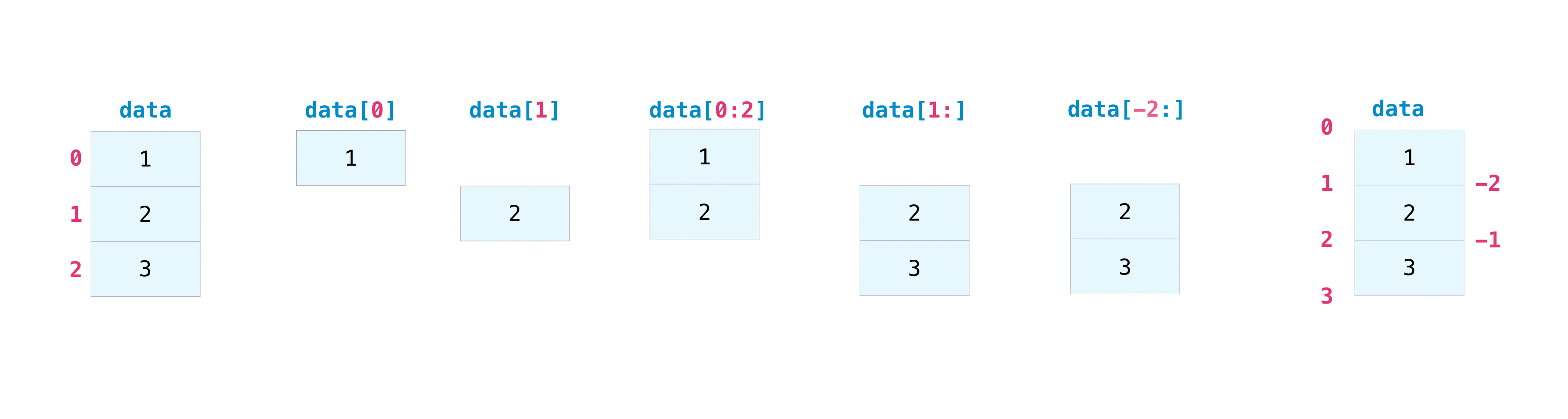You may want to take a section of your array or specific array elements to use in further analysis or additional operations. To do that, you’ll need to subset, slice, and/or index your arrays.

If you want to select values from your array that fulfill certain conditions, it’s straightforward with NumPy.

```>>> a = np.array([[1 , 2, 3, 4], [5, 6, 7, 8], [9, 10, 11, 12]])
```

You can easily print all of the values in the array that are less than 5.

```>>> print(a[a < 5])
[1 2 3 4]
```

You can also select, for example, numbers that are equal to or greater than 5, and use that condition to index an array.

```>>> five_up = (a >= 5)
>>> print(a[five_up])
[ 5  6  7  8  9 10 11 12]
```

You can select elements that are divisible by 2:

```>>> divisible_by_2 = a[a%2==0]
>>> print(divisible_by_2)
[ 2  4  6  8 10 12]
```

Or you can select elements that satisfy two conditions using the `&` and `|` operators:

```>>> c = a[(a > 2) & (a < 11)]
>>> print(c)
[ 3  4  5  6  7  8  9 10]
```

You can also make use of the logical operators & and | in order to return boolean values that specify whether or not the values in an array fulfill a certain condition. This can be useful with arrays that contain names or other categorical values.

```>>> five_up = (a > 5) | (a == 5)
>>> print(five_up)
[[False False False False]
[ True  True  True  True]
[ True  True  True True]]
```

You can also use `np.nonzero()` to select elements or indices from an array.

Starting with this array:

```>>> a = np.array([[1, 2, 3, 4], [5, 6, 7, 8], [9, 10, 11, 12]])
```

You can use `np.nonzero()` to print the indices of elements that are, for example, less than 5:

```>>> b = np.nonzero(a < 5)
>>> print(b)
(array([0, 0, 0, 0]), array([0, 1, 2, 3]))
```

In this example, a tuple of arrays was returned: one for each dimension. The first array represents the row indices where these values are found, and the second array represents the column indices where the values are found.

If you want to generate a list of coordinates where the elements exist, you can zip the arrays, iterate over the list of coordinates, and print them. For example:

```>>> list_of_coordinates= list(zip(b, b))

>>> for coord in list_of_coordinates:
...     print(coord)
(0, 0)
(0, 1)
(0, 2)
(0, 3)
```

You can also use `np.nonzero()` to print the elements in an array that are less than 5 with:

```>>> print(a[b])
[1 2 3 4]
```

If the element you’re looking for doesn’t exist in the array, then the returned array of indices will be empty. For example:

```>>> not_there = np.nonzero(a == 42)
>>> print(not_there)
(array([], dtype=int64), array([], dtype=int64))
```

Read more about using the nonzero function at: `nonzero`.

## How to create an array from existing data¶

This section covers `slicing and indexing`, `np.vstack()`, `np.hstack()`, `np.hsplit()`, `.view()`, `copy()`

You can easily use create a new array from a section of an existing array.

Let’s say you have this array:

```>>> a = np.array([1,  2,  3,  4,  5,  6,  7,  8,  9, 10])
```

You can create a new array from a section of your array any time by specifying where you want to slice your array.

```>>> arr1 = a[3:8]
>>> arr1
array([4, 5, 6, 7, 8])
```

Here, you grabbed a section of your array from index position 3 through index position 8.

You can also stack two existing arrays, both vertically and horizontally. Let’s say you have two arrays, `a1` and `a2`:

```>>> a1 = np.array([[1, 1],
...                [2, 2]])

>>> a2 = np.array([[3, 3],
...                [4, 4]])
```

You can stack them vertically with `vstack`:

```>>> np.vstack((a1, a2))
array([[1, 1],
[2, 2],
[3, 3],
[4, 4]])
```

Or stack them horizontally with `hstack`:

```>>> np.hstack((a1, a2))
array([[1, 1, 3, 3],
[2, 2, 4, 4]])
```

You can split an array into several smaller arrays using `hsplit`. You can specify either the number of equally shaped arrays to return or the columns after which the division should occur.

Let’s say you have this array:

```>>> x = np.arange(1, 25).reshape(2, 12)
>>> x
array([[ 1,  2,  3,  4,  5,  6,  7,  8,  9, 10, 11, 12],
[13, 14, 15, 16, 17, 18, 19, 20, 21, 22, 23, 24]])
```

If you wanted to split this array into three equally shaped arrays, you would run:

```>>> np.hsplit(x, 3)
[array([[1,  2,  3,  4],
[13, 14, 15, 16]]), array([[ 5,  6,  7,  8],
[17, 18, 19, 20]]), array([[ 9, 10, 11, 12],
[21, 22, 23, 24]])]
```

If you wanted to split your array after the third and fourth column, you’d run:

```>>> np.hsplit(x, (3, 4))
[array([[1, 2, 3],
[13, 14, 15]]), array([[ 4],
]), array([[ 5, 6, 7, 8, 9, 10, 11, 12],
[17, 18, 19, 20, 21, 22, 23, 24]])]
```

You can use the `view` method to create a new array object that looks at the same data as the original array (a shallow copy).

Views are an important NumPy concept! NumPy functions, as well as operations like indexing and slicing, will return views whenever possible. This saves memory and is faster (no copy of the data has to be made). However it’s important to be aware of this - modifying data in a view also modifies the original array!

Let’s say you create this array:

```>>> a = np.array([[1, 2, 3, 4], [5, 6, 7, 8], [9, 10, 11, 12]])
```

Now we create an array `b1` by slicing `a` and modify the first element of `b1`. This will modify the corresponding element in `a` as well!

```>>> b1 = a[0, :]
>>> b1
array([1, 2, 3, 4])
>>> b1 = 99
>>> b1
array([99,  2,  3,  4])
>>> a
array([[99,  2,  3,  4],
[ 5,  6,  7,  8],
[ 9, 10, 11, 12]])
```

Using the `copy` method will make a complete copy of the array and its data (a deep copy). To use this on your array, you could run:

```>>> b2 = a.copy()
```

## Basic array operations¶

This section covers addition, subtraction, multiplication, division, and more

Once you’ve created your arrays, you can start to work with them. Let’s say, for example, that you’ve created two arrays, one called “data” and one called “ones”You can add the arrays together with the plus sign.

```>>> data = np.array([1, 2])
>>> ones = np.ones(2, dtype=int)
>>> data + ones
array([2, 3])
```You can, of course, do more than just addition!

```>>> data - ones
array([0, 1])
>>> data * data
array([1, 4])
>>> data / data
array([1., 1.])
```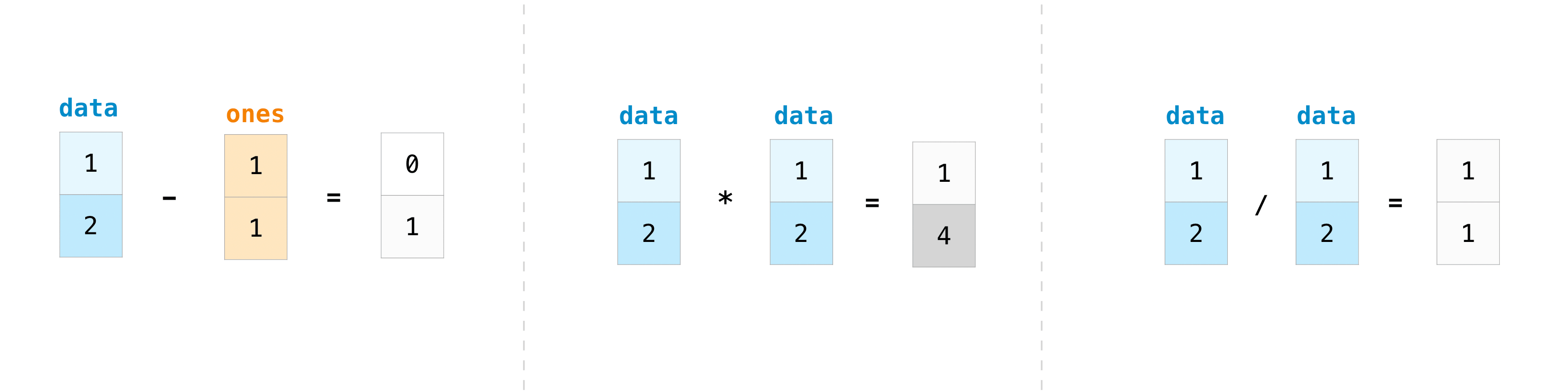Basic operations are simple with NumPy. If you want to find the sum of the elements in an array, you’d use `sum()`. This works for 1D arrays, 2D arrays, and arrays in higher dimensions.

```>>> a = np.array([1, 2, 3, 4])

>>> a.sum()
10
```

To add the rows or the columns in a 2D array, you would specify the axis.

```>>> b = np.array([[1, 1], [2, 2]])
```

You can sum the rows with:

```>>> b.sum(axis=0)
array([3, 3])
```

You can sum the columns with:

```>>> b.sum(axis=1)
array([2, 4])
```

There are times when you might want to carry out an operation between an array and a single number (also called an operation between a vector and a scalar) or between arrays of two different sizes. For example, your array (we’ll call it “data”) might contain information about distance in miles but you want to convert the information to kilometers. You can perform this operation with:

```>>> data = np.array([1.0, 2.0])
>>> data * 1.6
array([1.6, 3.2])
```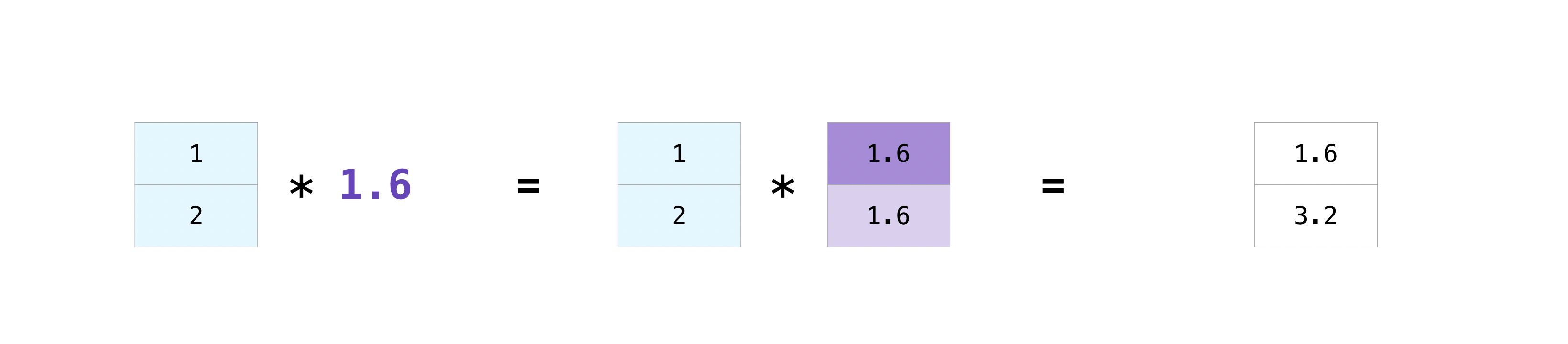NumPy understands that the multiplication should happen with each cell. That concept is called broadcasting. Broadcasting is a mechanism that allows NumPy to perform operations on arrays of different shapes. The dimensions of your array must be compatible, for example, when the dimensions of both arrays are equal or when one of them is 1. If the dimensions are not compatible, you will get a `ValueError`.

## More useful array operations¶

This section covers maximum, minimum, sum, mean, product, standard deviation, and more

NumPy also performs aggregation functions. In addition to `min`, `max`, and `sum`, you can easily run `mean` to get the average, `prod` to get the result of multiplying the elements together, `std` to get the standard deviation, and more.

```>>> data.max()
2.0
>>> data.min()
1.0
>>> data.sum()
3.0
```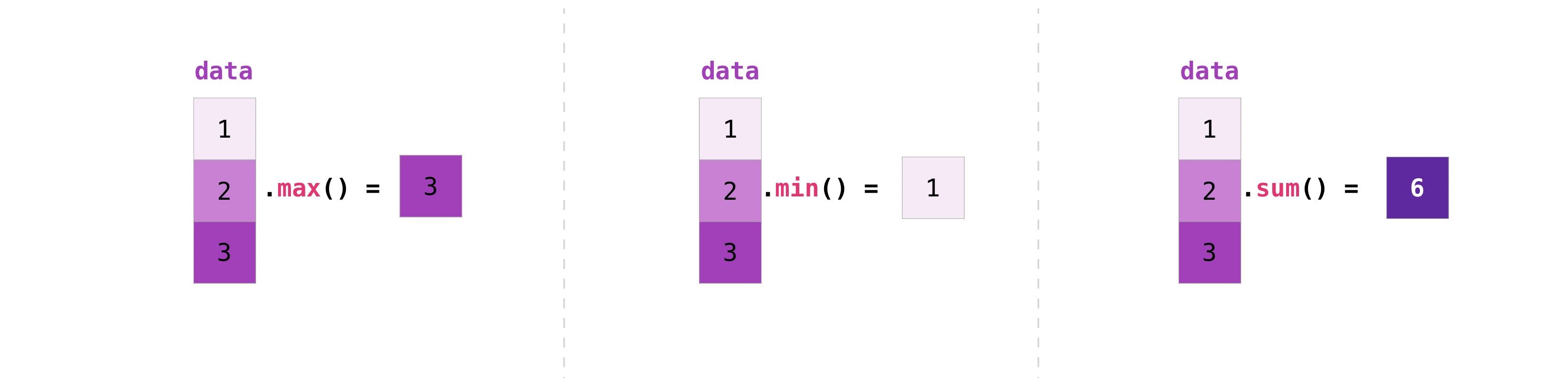```>>> a = np.array([[0.45053314, 0.17296777, 0.34376245, 0.5510652],
...               [0.54627315, 0.05093587, 0.40067661, 0.55645993],
...               [0.12697628, 0.82485143, 0.26590556, 0.56917101]])
```

It’s very common to want to aggregate along a row or column. By default, every NumPy aggregation function will return the aggregate of the entire array. To find the sum or the minimum of the elements in your array, run:

```>>> a.sum()
4.8595784
```

Or:

```>>> a.min()
0.05093587
```

You can specify on which axis you want the aggregation function to be computed. For example, you can find the minimum value within each column by specifying `axis=0`.

```>>> a.min(axis=0)
array([0.12697628, 0.05093587, 0.26590556, 0.5510652 ])
```

The four values listed above correspond to the number of columns in your array. With a four-column array, you will get four values as your result.

## Creating matrices¶

You can pass Python lists of lists to create a 2-D array (or “matrix”) to represent them in NumPy.

```>>> data = np.array([[1, 2], [3, 4]])
>>> data
array([[1, 2],
[3, 4]])
```Indexing and slicing operations are useful when you’re manipulating matrices:

```>>> data[0, 1]
2
>>> data[1:3]
array([[3, 4]])
>>> data[0:2, 0]
array([1, 3])
```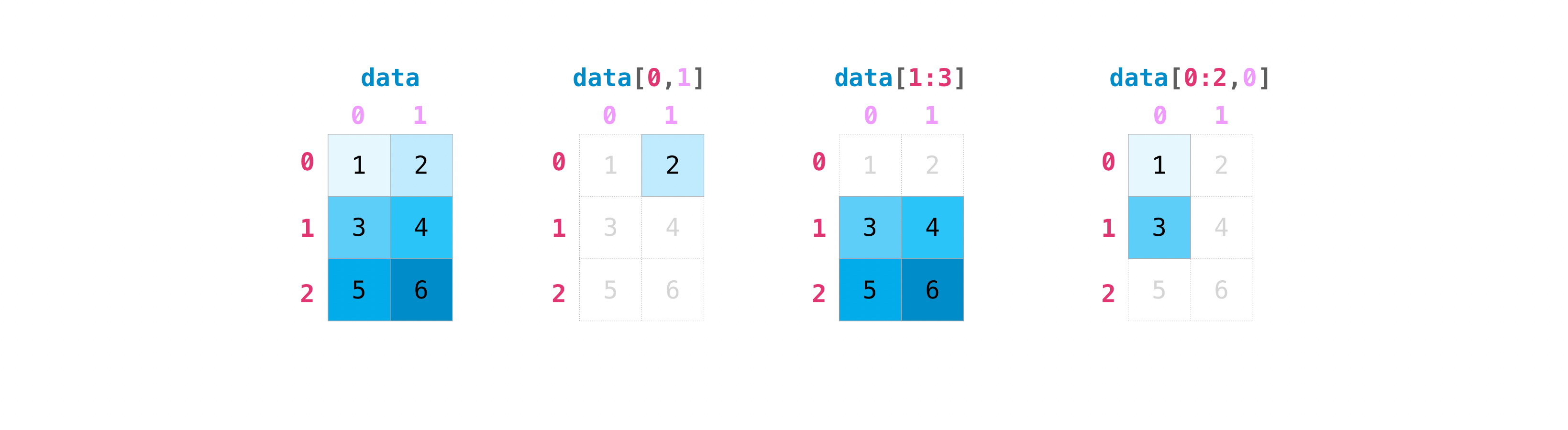You can aggregate matrices the same way you aggregated vectors:

```>>> data.max()
4
>>> data.min()
1
>>> data.sum()
10
```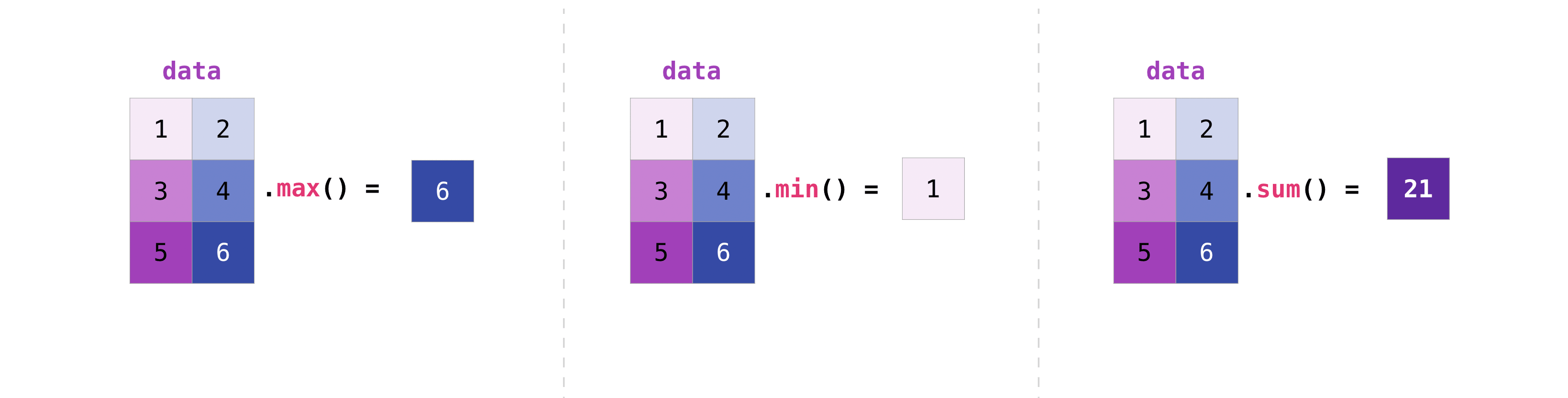You can aggregate all the values in a matrix and you can aggregate them across columns or rows using the `axis` parameter:

```>>> data.max(axis=0)
array([3, 4])
>>> data.max(axis=1)
array([2, 4])
```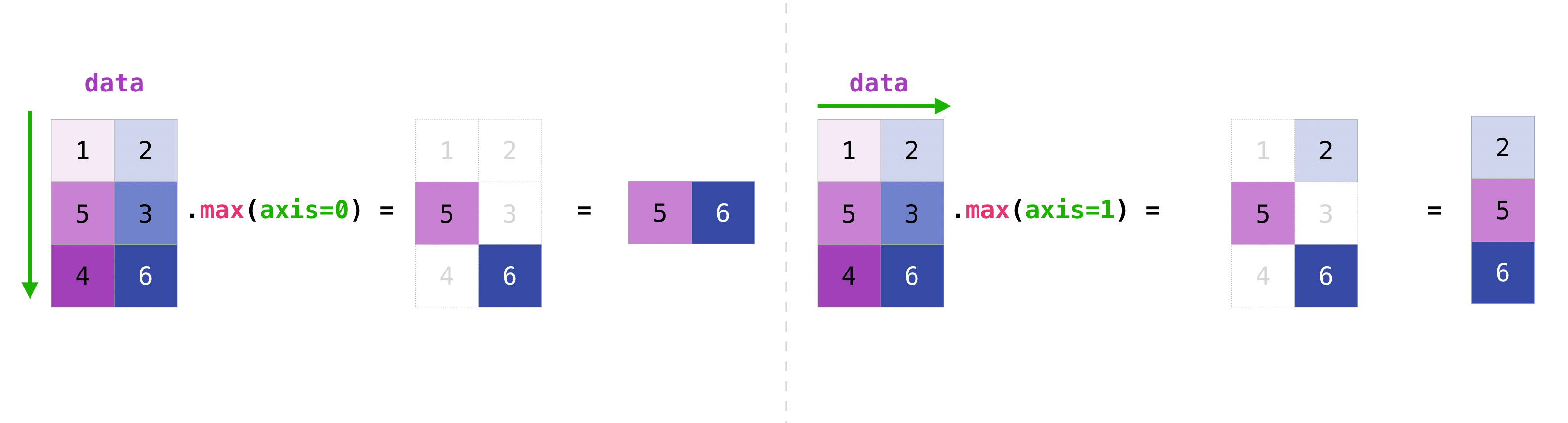Once you’ve created your matrices, you can add and multiply them using arithmetic operators if you have two matrices that are the same size.

```>>> data = np.array([[1, 2], [3, 4]])
>>> ones = np.array([[1, 1], [1, 1]])
>>> data + ones
array([[2, 3],
[4, 5]])
```You can do these arithmetic operations on matrices of different sizes, but only if one matrix has only one column or one row. In this case, NumPy will use its broadcast rules for the operation.

```>>> data = np.array([[1, 2], [3, 4], [5, 6]])
>>> ones_row = np.array([[1, 1]])
>>> data + ones_row
array([[2, 3],
[4, 5],
[6, 7]])
```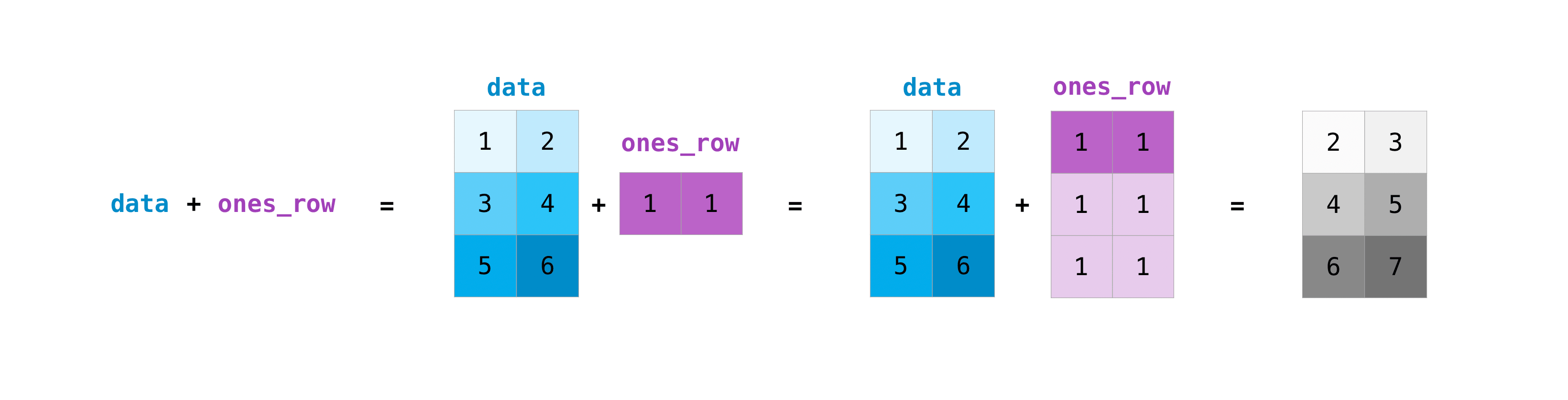Be aware that when NumPy prints N-dimensional arrays, the last axis is looped over the fastest while the first axis is the slowest. For instance:

```>>> np.ones((4, 3, 2))
array([[[1., 1.],
[1., 1.],
[1., 1.]],

[[1., 1.],
[1., 1.],
[1., 1.]],

[[1., 1.],
[1., 1.],
[1., 1.]],

[[1., 1.],
[1., 1.],
[1., 1.]]])
```

There are often instances where we want NumPy to initialize the values of an array. NumPy offers functions like `ones()` and `zeros()`, and the `random.Generator` class for random number generation for that. All you need to do is pass in the number of elements you want it to generate:

```>>> np.ones(3)
array([1., 1., 1.])
>>> np.zeros(3)
array([0., 0., 0.])
# the simplest way to generate random numbers
>>> rng = np.random.default_rng(0)
>>> rng.random(3)
array([0.63696169, 0.26978671, 0.04097352])
```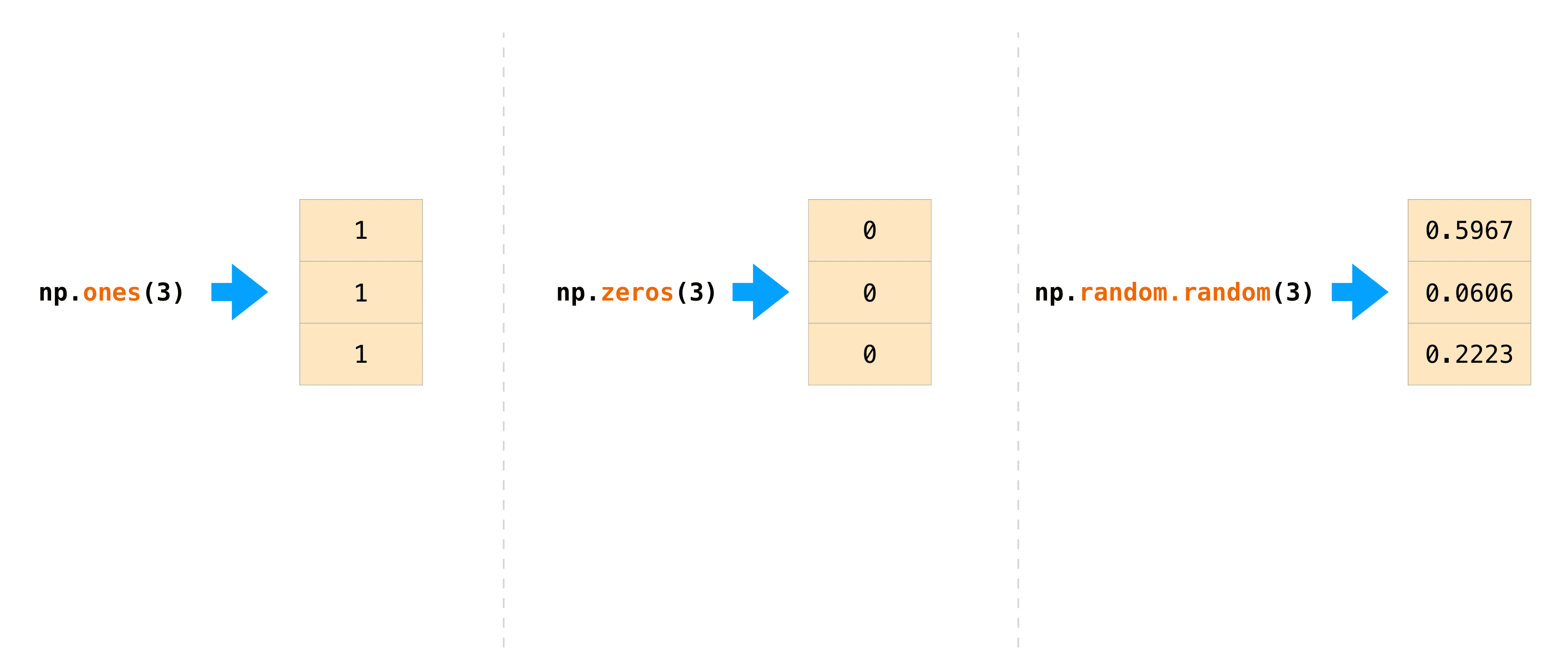You can also use `ones()`, `zeros()`, and `random()` to create a 2D array if you give them a tuple describing the dimensions of the matrix:

```>>> np.ones((3, 2))
array([[1., 1.],
[1., 1.],
[1., 1.]])
>>> np.zeros((3, 2))
array([[0., 0.],
[0., 0.],
[0., 0.]])
>>> rng.random((3, 2))
array([[0.01652764, 0.81327024],
[0.91275558, 0.60663578],
[0.72949656, 0.54362499]])  # may vary
```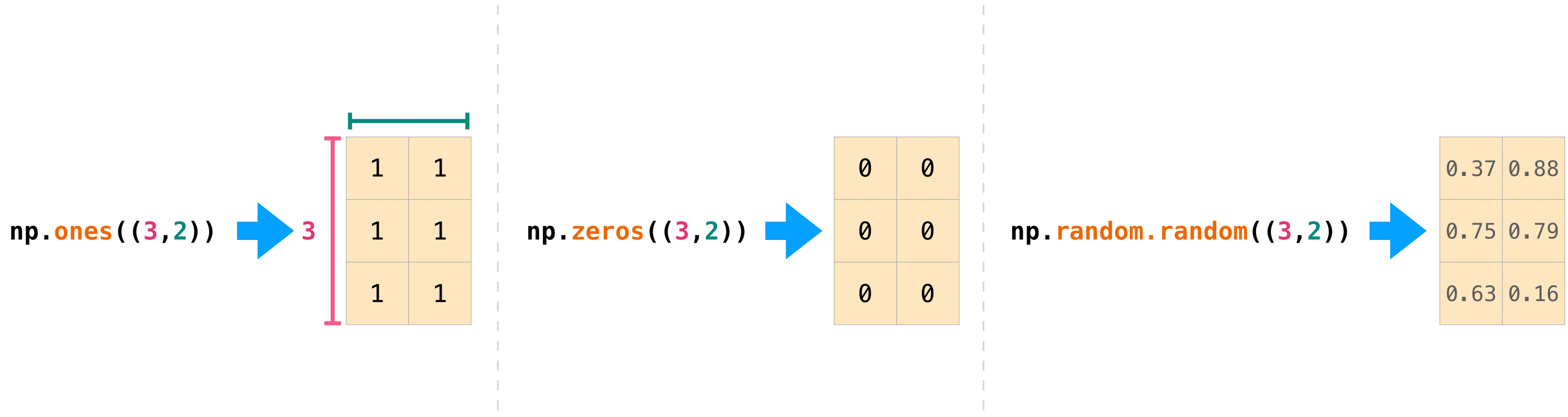Read more about creating arrays, filled with `0`’s, `1`’s, other values or uninitialized, at array creation routines.

## Generating random numbers¶

The use of random number generation is an important part of the configuration and evaluation of many numerical and machine learning algorithms. Whether you need to randomly initialize weights in an artificial neural network, split data into random sets, or randomly shuffle your dataset, being able to generate random numbers (actually, repeatable pseudo-random numbers) is essential.

With `Generator.integers`, you can generate random integers from low (remember that this is inclusive with NumPy) to high (exclusive). You can set `endpoint=True` to make the high number inclusive.

You can generate a 2 x 4 array of random integers between 0 and 4 with:

```>>> rng.integers(5, size=(2, 4))
array([[2, 1, 1, 0],
[0, 0, 0, 4]])  # may vary
```

## How to get unique items and counts¶

This section covers `np.unique()`

You can find the unique elements in an array easily with `np.unique`.

```>>> a = np.array([11, 11, 12, 13, 14, 15, 16, 17, 12, 13, 11, 14, 18, 19, 20])
```

you can use `np.unique` to print the unique values in your array:

```>>> unique_values = np.unique(a)
>>> print(unique_values)
[11 12 13 14 15 16 17 18 19 20]
```

To get the indices of unique values in a NumPy array (an array of first index positions of unique values in the array), just pass the `return_index` argument in `np.unique()` as well as your array.

```>>> unique_values, indices_list = np.unique(a, return_index=True)
>>> print(indices_list)
[ 0  2  3  4  5  6  7 12 13 14]
```

You can pass the `return_counts` argument in `np.unique()` along with your array to get the frequency count of unique values in a NumPy array.

```>>> unique_values, occurrence_count = np.unique(a, return_counts=True)
>>> print(occurrence_count)
[3 2 2 2 1 1 1 1 1 1]
```

This also works with 2D arrays! If you start with this array:

```>>> a_2d = np.array([[1, 2, 3, 4], [5, 6, 7, 8], [9, 10, 11, 12], [1, 2, 3, 4]])
```

You can find unique values with:

```>>> unique_values = np.unique(a_2d)
>>> print(unique_values)
[ 1  2  3  4  5  6  7  8  9 10 11 12]
```

If the axis argument isn’t passed, your 2D array will be flattened.

If you want to get the unique rows or columns, make sure to pass the `axis` argument. To find the unique rows, specify `axis=0` and for columns, specify `axis=1`.

```>>> unique_rows = np.unique(a_2d, axis=0)
>>> print(unique_rows)
[[ 1  2  3  4]
[ 5  6  7  8]
[ 9 10 11 12]]
```

To get the unique rows, index position, and occurrence count, you can use:

```>>> unique_rows, indices, occurrence_count = np.unique(
...      a_2d, axis=0, return_counts=True, return_index=True)
>>> print(unique_rows)
[[ 1  2  3  4]
[ 5  6  7  8]
[ 9 10 11 12]]
>>> print(indices)
[0 1 2]
>>> print(occurrence_count)
[2 1 1]
```

To learn more about finding the unique elements in an array, see `unique`.

## Transposing and reshaping a matrix¶

This section covers `arr.reshape()`, `arr.transpose()`, `arr.T()`

It’s common to need to transpose your matrices. NumPy arrays have the property `T` that allows you to transpose a matrix.You may also need to switch the dimensions of a matrix. This can happen when, for example, you have a model that expects a certain input shape that is different from your dataset. This is where the `reshape` method can be useful. You simply need to pass in the new dimensions that you want for the matrix.

```>>> data.reshape(2, 3)
array([[1, 2, 3],
[4, 5, 6]])
>>> data.reshape(3, 2)
array([[1, 2],
[3, 4],
[5, 6]])
```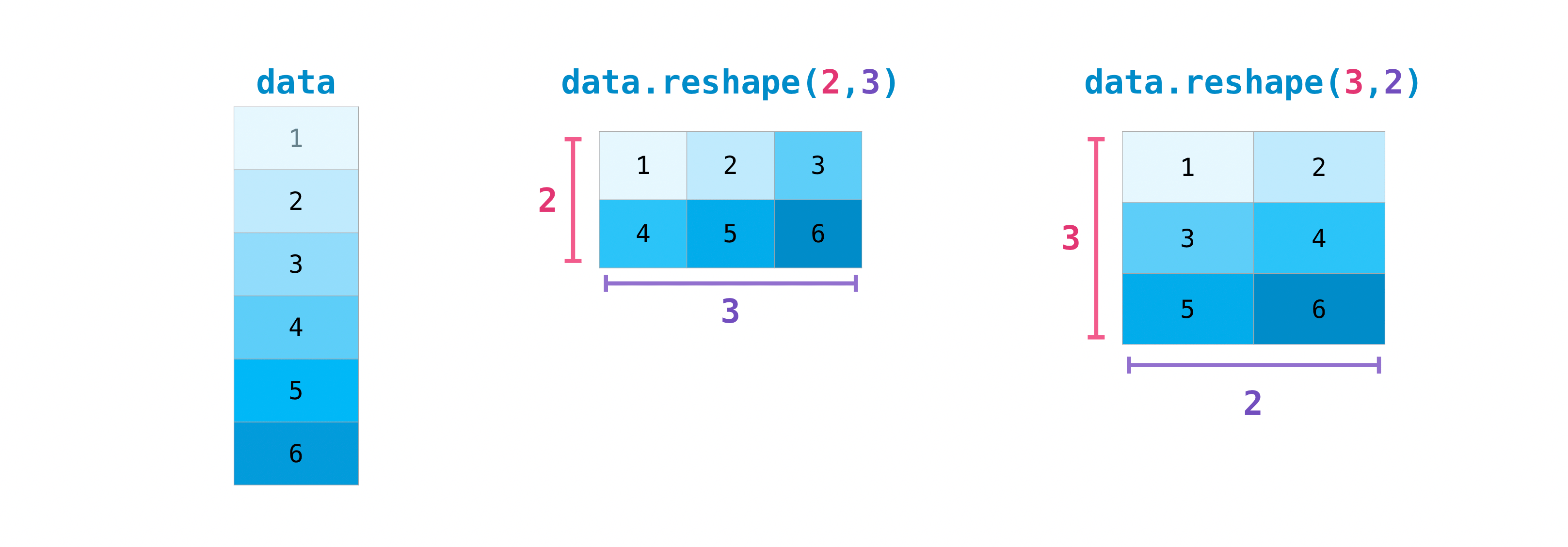You can also use `.transpose` to reverse or change the axes of an array according to the values you specify.

```>>> arr = np.arange(6).reshape((2, 3))
>>> arr
array([[0, 1, 2],
[3, 4, 5]])
```

You can transpose your array with `arr.transpose()`.

```>>> arr.transpose()
array([[0, 3],
[1, 4],
[2, 5]])
```

To learn more about transposing and reshaping arrays, see `transpose` and `reshape`.

## How to reverse an array¶

This section covers `np.flip`

NumPy’s `np.flip()` function allows you to flip, or reverse, the contents of an array along an axis. When using `np.flip`, specify the array you would like to reverse and the axis. If you don’t specify the axis, NumPy will reverse the contents along all of the axes of your input array.

Reversing a 1D array

If you begin with a 1D array like this one:

```>>> arr = np.array([1, 2, 3, 4, 5, 6, 7, 8])
```

You can reverse it with:

```>>> reversed_arr = np.flip(arr)
```

If you want to print your reversed array, you can run:

```>>> print('Reversed Array: ', reversed_arr)
Reversed Array:  [8 7 6 5 4 3 2 1]
```

Reversing a 2D array

A 2D array works much the same way.

```>>> arr_2d = np.array([[1, 2, 3, 4], [5, 6, 7, 8], [9, 10, 11, 12]])
```

You can reverse the content in all of the rows and all of the columns with:

```>>> reversed_arr = np.flip(arr_2d)
>>> print(reversed_arr)
[[12 11 10  9]
[ 8  7  6  5]
[ 4  3  2  1]]
```

You can easily reverse only the rows with:

```>>> reversed_arr_rows = np.flip(arr_2d, axis=0)
>>> print(reversed_arr_rows)
[[ 9 10 11 12]
[ 5  6  7  8]
[ 1  2  3  4]]
```

Or reverse only the columns with:

```>>> reversed_arr_columns = np.flip(arr_2d, axis=1)
>>> print(reversed_arr_columns)
[[ 4  3  2  1]
[ 8  7  6  5]
[12 11 10  9]]
```

You can also reverse the contents of only one column or row. For example, you can reverse the contents of the row at index position 1 (the second row):

```>>> arr_2d = np.flip(arr_2d)
>>> print(arr_2d)
[[ 1  2  3  4]
[ 8  7  6  5]
[ 9 10 11 12]]
```

You can also reverse the column at index position 1 (the second column):

```>>> arr_2d[:,1] = np.flip(arr_2d[:,1])
>>> print(arr_2d)
[[ 1 10  3  4]
[ 8  7  6  5]
[ 9  2 11 12]]
```

Read more about reversing arrays at `flip`.

## Reshaping and flattening multidimensional arrays¶

This section covers `.flatten()`, `ravel()`

There are two popular ways to flatten an array: `.flatten()` and `.ravel()`. The primary difference between the two is that the new array created using `ravel()` is actually a reference to the parent array (i.e., a “view”). This means that any changes to the new array will affect the parent array as well. Since `ravel` does not create a copy, it’s memory efficient.

```>>> x = np.array([[1 , 2, 3, 4], [5, 6, 7, 8], [9, 10, 11, 12]])
```

You can use `flatten` to flatten your array into a 1D array.

```>>> x.flatten()
array([ 1,  2,  3,  4,  5,  6,  7,  8,  9, 10, 11, 12])
```

When you use `flatten`, changes to your new array won’t change the parent array.

For example:

```>>> a1 = x.flatten()
>>> a1 = 99
>>> print(x)  # Original array
[[ 1  2  3  4]
[ 5  6  7  8]
[ 9 10 11 12]]
>>> print(a1)  # New array
[99  2  3  4  5  6  7  8  9 10 11 12]
```

But when you use `ravel`, the changes you make to the new array will affect the parent array.

For example:

```>>> a2 = x.ravel()
>>> a2 = 98
>>> print(x)  # Original array
[[98  2  3  4]
[ 5  6  7  8]
[ 9 10 11 12]]
>>> print(a2)  # New array
[98  2  3  4  5  6  7  8  9 10 11 12]
```

Read more about `flatten` at `ndarray.flatten` and `ravel` at `ravel`.

This section covers `help()`, `?`, `??`

When it comes to the data science ecosystem, Python and NumPy are built with the user in mind. One of the best examples of this is the built-in access to documentation. Every object contains the reference to a string, which is known as the docstring. In most cases, this docstring contains a quick and concise summary of the object and how to use it. Python has a built-in `help()` function that can help you access this information. This means that nearly any time you need more information, you can use `help()` to quickly find the information that you need.

For example:

```>>> help(max)
Help on built-in function max in module builtins:

max(...)
max(iterable, *[, default=obj, key=func]) -> value
max(arg1, arg2, *args, *[, key=func]) -> value

With a single iterable argument, return its biggest item. The
default keyword-only argument specifies an object to return if
the provided iterable is empty.
With two or more arguments, return the largest argument.
```

Because access to additional information is so useful, IPython uses the `?` character as a shorthand for accessing this documentation along with other relevant information. IPython is a command shell for interactive computing in multiple languages. You can find more information about IPython here.

For example:

```In : max?
max(iterable, *[, default=obj, key=func]) -> value
max(arg1, arg2, *args, *[, key=func]) -> value

With a single iterable argument, return its biggest item. The
default keyword-only argument specifies an object to return if
the provided iterable is empty.
With two or more arguments, return the largest argument.
Type:      builtin_function_or_method
```

You can even use this notation for object methods and objects themselves.

Let’s say you create this array:

```>>> a = np.array([1, 2, 3, 4, 5, 6])
```

Then you can obtain a lot of useful information (first details about `a` itself, followed by the docstring of `ndarray` of which `a` is an instance):

```In : a?
Type:            ndarray
String form:     [1 2 3 4 5 6]
Length:          6
File:            ~/anaconda3/lib/python3.7/site-packages/numpy/__init__.py
Docstring:       <no docstring>
Class docstring:
ndarray(shape, dtype=float, buffer=None, offset=0,
strides=None, order=None)

An array object represents a multidimensional, homogeneous array
of fixed-size items.  An associated data-type object describes the
format of each element in the array (its byte-order, how many bytes it
occupies in memory, whether it is an integer, a floating point number,
or something else, etc.)

Arrays should be constructed using `array`, `zeros` or `empty` (refer
to the See Also section below).  The parameters given here refer to
a low-level method (`ndarray(...)`) for instantiating an array.

methods and attributes of an array.

Parameters
----------
(for the __new__ method; see Notes below)

shape : tuple of ints
Shape of created array.
...
```

This also works for functions and other objects that you create. Just remember to include a docstring with your function using a string literal (`""" """` or `''' '''` around your documentation).

For example, if you create this function:

```>>> def double(a):
...   '''Return a * 2'''
...   return a * 2
```

You can obtain information about the function:

```In : double?
Signature: double(a)
Docstring: Return a * 2
Type:      function
```

You can reach another level of information by reading the source code of the object you’re interested in. Using a double question mark (`??`) allows you to access the source code.

For example:

```In : double??
Signature: double(a)
Source:
def double(a):
'''Return a * 2'''
return a * 2
Type:      function
```

If the object in question is compiled in a language other than Python, using `??` will return the same information as `?`. You’ll find this with a lot of built-in objects and types, for example:

```In : len?
Signature: len(obj, /)
Docstring: Return the number of items in a container.
Type:      builtin_function_or_method
```

and :

```In : len??
Signature: len(obj, /)
Docstring: Return the number of items in a container.
Type:      builtin_function_or_method
```

have the same output because they were compiled in a programming language other than Python.

## Working with mathematical formulas¶

The ease of implementing mathematical formulas that work on arrays is one of the things that make NumPy so widely used in the scientific Python community.

For example, this is the mean square error formula (a central formula used in supervised machine learning models that deal with regression):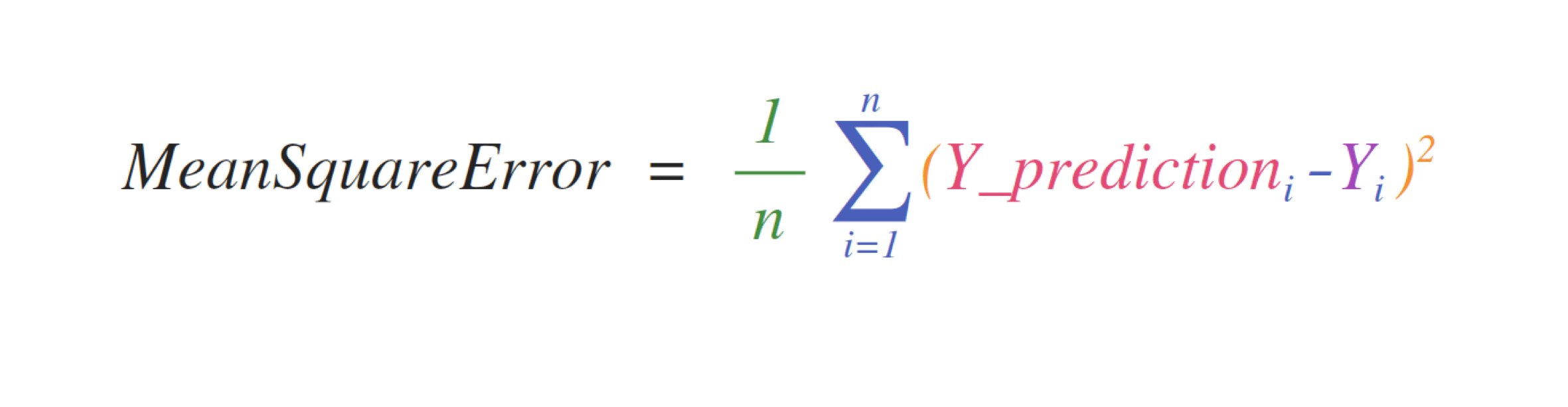Implementing this formula is simple and straightforward in NumPy: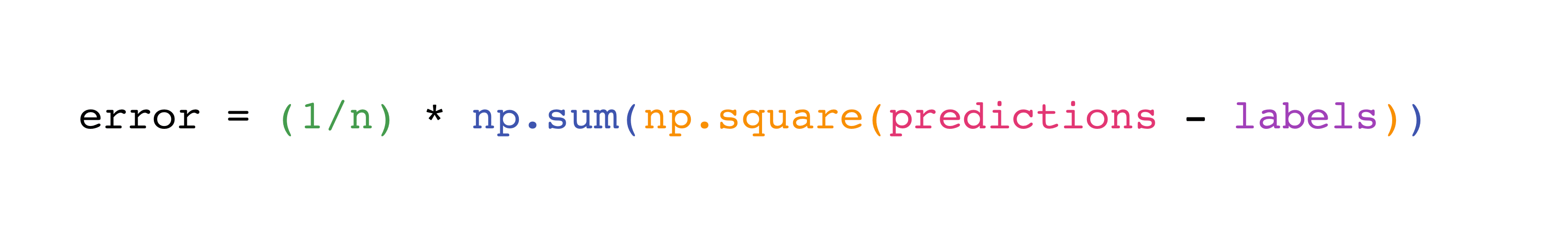What makes this work so well is that `predictions` and `labels` can contain one or a thousand values. They only need to be the same size.

You can visualize it this way: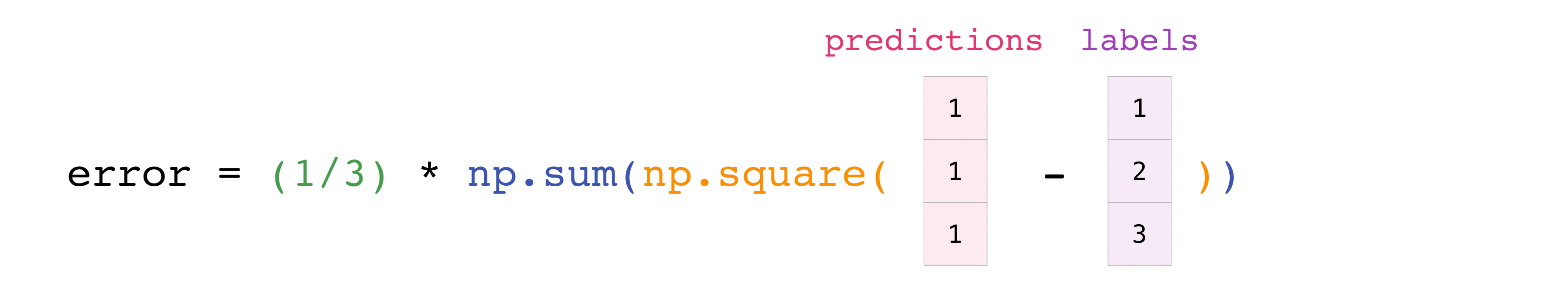In this example, both the predictions and labels vectors contain three values, meaning `n` has a value of three. After we carry out subtractions the values in the vector are squared. Then NumPy sums the values, and your result is the error value for that prediction and a score for the quality of the model.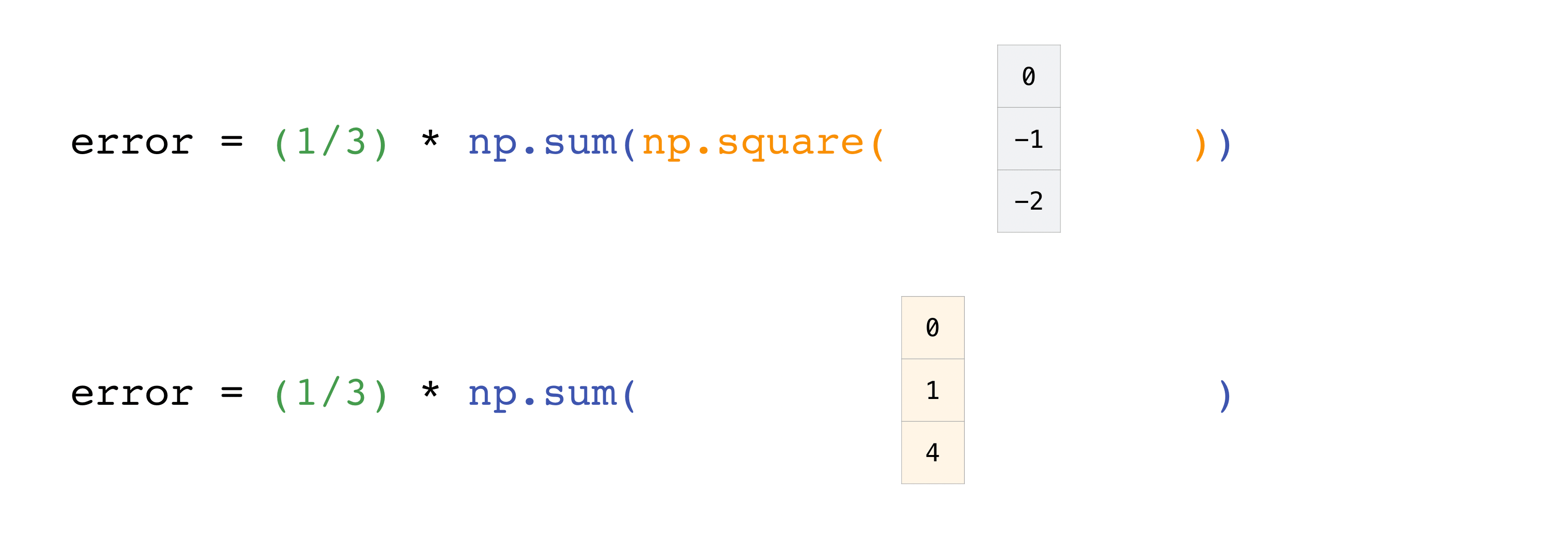## How to save and load NumPy objects¶

This section covers `np.save`, `np.savez`, `np.savetxt`, `np.load`, `np.loadtxt`

You will, at some point, want to save your arrays to disk and load them back without having to re-run the code. Fortunately, there are several ways to save and load objects with NumPy. The ndarray objects can be saved to and loaded from the disk files with `loadtxt` and `savetxt` functions that handle normal text files, `load` and `save` functions that handle NumPy binary files with a .npy file extension, and a `savez` function that handles NumPy files with a .npz file extension.

The .npy and .npz files store data, shape, dtype, and other information required to reconstruct the ndarray in a way that allows the array to be correctly retrieved, even when the file is on another machine with different architecture.

If you want to store a single ndarray object, store it as a .npy file using `np.save`. If you want to store more than one ndarray object in a single file, save it as a .npz file using `np.savez`. You can also save several arrays into a single file in compressed npz format with `savez_compressed`.

It’s easy to save and load and array with `np.save()`. Just make sure to specify the array you want to save and a file name. For example, if you create this array:

```>>> a = np.array([1, 2, 3, 4, 5, 6])
```

You can save it as “filename.npy” with:

```>>> np.save('filename', a)
```

You can use `np.load()` to reconstruct your array.

```>>> b = np.load('filename.npy')
```

If you want to check your array, you can run::

```>>> print(b)
[1 2 3 4 5 6]
```

You can save a NumPy array as a plain text file like a .csv or .txt file with `np.savetxt`.

For example, if you create this array:

```>>> csv_arr = np.array([1, 2, 3, 4, 5, 6, 7, 8])
```

You can easily save it as a .csv file with the name “new_file.csv” like this:

```>>> np.savetxt('new_file.csv', csv_arr)
```

You can quickly and easily load your saved text file using `loadtxt()`:

```>>> np.loadtxt('new_file.csv')
array([1., 2., 3., 4., 5., 6., 7., 8.])
```

The `savetxt()` and `loadtxt()` functions accept additional optional parameters such as header, footer, and delimiter. While text files can be easier for sharing, .npy and .npz files are smaller and faster to read. If you need more sophisticated handling of your text file (for example, if you need to work with lines that contain missing values), you will want to use the `genfromtxt` function.

With `savetxt`, you can specify headers, footers, comments, and more.

## Importing and exporting a CSV¶

It’s simple to read in a CSV that contains existing information. The best and easiest way to do this is to use Pandas.

```>>> import pandas as pd

>>> # If all of your columns are the same type:
>>> print(x)
[['Billie Holiday' 'Jazz' 1300000 27000000]
['Jimmie Hendrix' 'Rock' 2700000 70000000]
['Miles Davis' 'Jazz' 1500000 48000000]
['SIA' 'Pop' 2000000 74000000]]

>>> # You can also simply select the columns you need:
>>> x = pd.read_csv('music.csv', usecols=['Artist', 'Plays']).values
>>> print(x)
[['Billie Holiday' 27000000]
['Jimmie Hendrix' 70000000]
['Miles Davis' 48000000]
['SIA' 74000000]]
```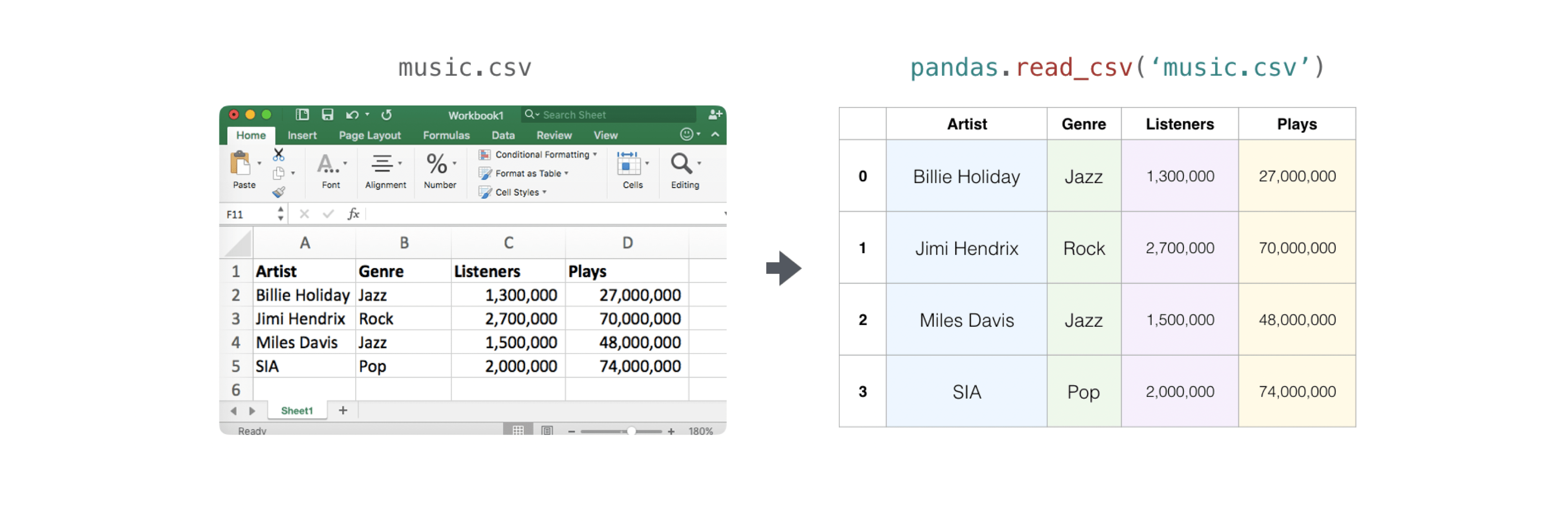It’s simple to use Pandas in order to export your array as well. If you are new to NumPy, you may want to create a Pandas dataframe from the values in your array and then write the data frame to a CSV file with Pandas.

If you created this array “a”

```>>> a = np.array([[-2.58289208,  0.43014843, -1.24082018, 1.59572603],
...               [ 0.99027828, 1.17150989,  0.94125714, -0.14692469],
...               [ 0.76989341,  0.81299683, -0.95068423, 0.11769564],
...               [ 0.20484034,  0.34784527,  1.96979195, 0.51992837]])
```

You could create a Pandas dataframe

```>>> df = pd.DataFrame(a)
>>> print(df)
0         1         2         3
0 -2.582892  0.430148 -1.240820  1.595726
1  0.990278  1.171510  0.941257 -0.146925
2  0.769893  0.812997 -0.950684  0.117696
3  0.204840  0.347845  1.969792  0.519928
```

You can easily save your dataframe with:

```>>> df.to_csv('pd.csv')
```

```>>> data = pd.read_csv('pd.csv')
```You can also save your array with the NumPy `savetxt` method.

```>>> np.savetxt('np.csv', a, fmt='%.2f', delimiter=',', header='1,  2,  3,  4')
```

If you’re using the command line, you can read your saved CSV any time with a command such as:

```\$ cat np.csv
#  1,  2,  3,  4
-2.58,0.43,-1.24,1.60
0.99,1.17,0.94,-0.15
0.77,0.81,-0.95,0.12
0.20,0.35,1.97,0.52
```

Or you can open the file any time with a text editor!

If you’re interested in learning more about Pandas, take a look at the official Pandas documentation. Learn how to install Pandas with the official Pandas installation information.

## Plotting arrays with Matplotlib¶

If you need to generate a plot for your values, it’s very simple with Matplotlib.

For example, you may have an array like this one:

```>>> a = np.array([2, 1, 5, 7, 4, 6, 8, 14, 10, 9, 18, 20, 22])
```

If you already have Matplotlib installed, you can import it with:

```>>> import matplotlib.pyplot as plt

# If you're using Jupyter Notebook, you may also want to run the following
# line of code to display your code in the notebook:

%matplotlib inline
```

All you need to do to plot your values is run:

```>>> plt.plot(a)

# If you are running from a command line, you may need to do this:
# >>> plt.show()
```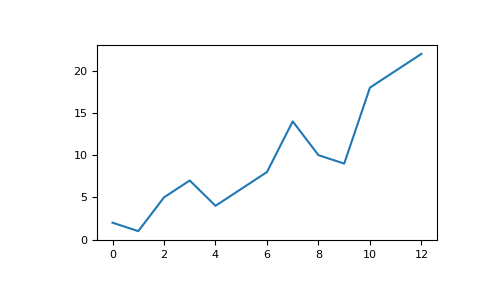For example, you can plot a 1D array like this:

```>>> x = np.linspace(0, 5, 20)
>>> y = np.linspace(0, 10, 20)
>>> plt.plot(x, y, 'purple') # line
>>> plt.plot(x, y, 'o')      # dots
```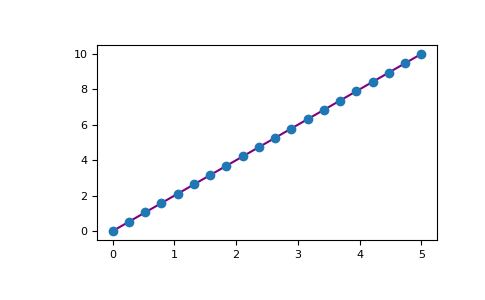With Matplotlib, you have access to an enormous number of visualization options.

```>>> from mpl_toolkits.mplot3d import Axes3D
>>> fig = plt.figure()
>>> ax = Axes3D(fig)
>>> X = np.arange(-5, 5, 0.15)
>>> Y = np.arange(-5, 5, 0.15)
>>> X, Y = np.meshgrid(X, Y)
>>> R = np.sqrt(X**2 + Y**2)
>>> Z = np.sin(R)

>>> ax.plot_surface(X, Y, Z, rstride=1, cstride=1, cmap='viridis')
```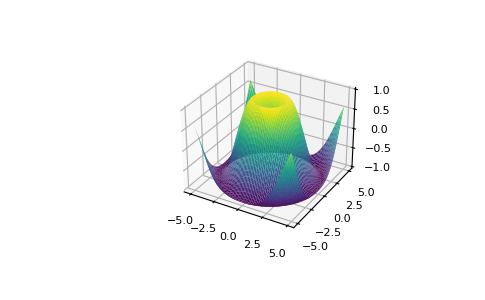To read more about Matplotlib and what it can do, take a look at the official documentation. For directions regarding installing Matplotlib, see the official installation section.

Image credits: Jay Alammar http://jalammar.github.io/